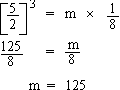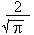Support the Monkey! Tell All your Friends and TeachersHome MonkeyNotes Printable Notes Digital Library Study Guides Study Smart Parents Tips College Planning Test Prep Fun Zone Help / FAQ How to Cite New Title Request

 Section 2 : Mathematical Reasoning If 55055 + x = 55555 x = 500 then, 55,555 - 100x = 55,555 - 50000 = 5555 Ans : C [(3y2) x2]4 = (3y2)4 (x2 )4 [Using law of indices (xm)n = xmn ] = 34y8x8 = 81y8x8   Ans : DAns : B Let the radius of the circle be r. Let the side of the square be x. Area of circle = p r2 Area of squares = x2 p r2 = x2 Since x2 = 4 p r2 = 4 r =C = 2 p r = 2 p ´= 4 Ö p Ans : A Index Test 3 Section 1: Verbal Reasoning Section 2: Mathematical Reasoning Section 3: Verbal Reasoning Section 4: Mathematical Reasoning Section 5: Reading Comprehension Section 6: Mathematical Reasoning Section 7: Mathematical Reasoning Answer Key To Test 3 Answer Explanation To Test 3 Section 1: Verbal Reasoning Section 2: Mathematical Reasoning Section 3: Verbal Reasoning Section 4: Mathematical Reasoning Section 5: Reading Comprehension Section 6: Mathematical Reasoning Section 7: Mathematical Reasoning Test 4
 Search: All Products Books Popular Music Classical Music Video DVD Toys & Games Electronics Software Tools & Hardware Outdoor Living Kitchen & Housewares Camera & Photo Cell Phones Keywords: